Logic

# Rook Synergy

In the following position, what is the smallest number of moves White can make to force checkmate?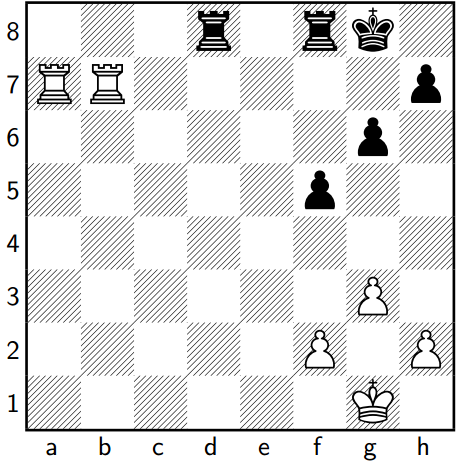In the following position, what is the smallest number of moves White can make to force checkmate?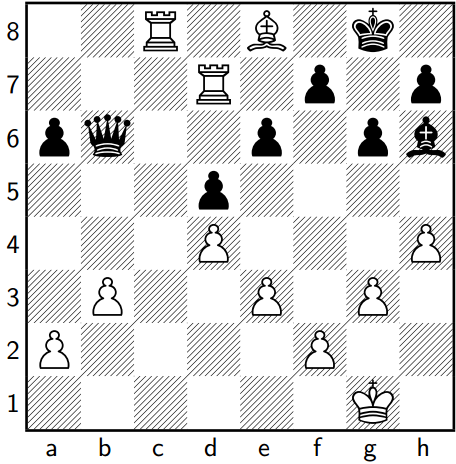In the following position, what is the smallest number of moves White can make to force checkmate?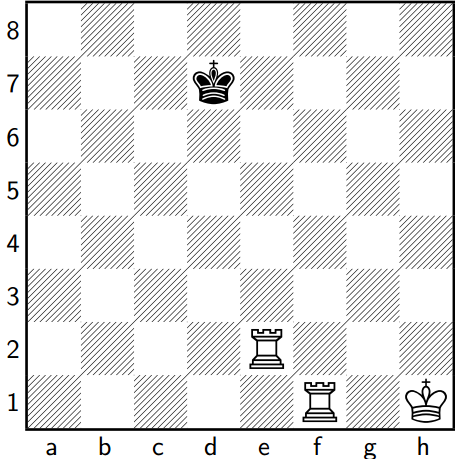In the following position, what is the smallest number of moves White can make to force checkmate?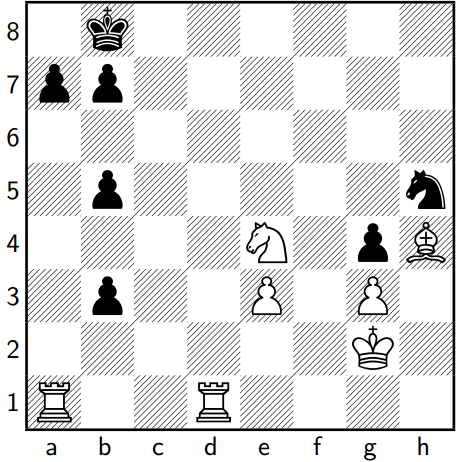In the following position, what is White's strongest move?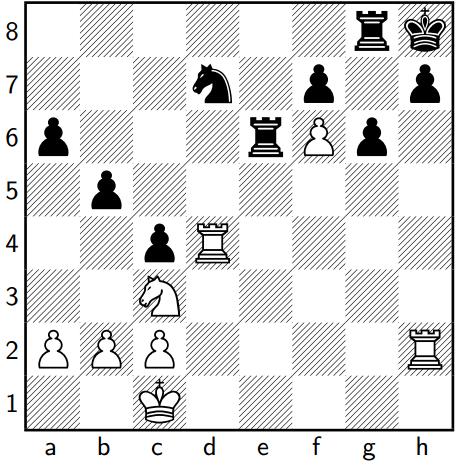Input the sum of the coordinates of the target square, where a=1, b=2, ..., h=8. For example, an answer of d5 would be encoded as 9 (d=4, and 4+5=9).

×